Online Store Directory

Spiritual Meditations and Exercises

Who We Are and What We Teach

Brotherhood of Light Lessons: Course Books on Astrology, Alchemy and Tarot

Astrology Software

Calendar of Activities

Astrological Sunday Services

Church of Light TV

Classes

Quarterly

Support UsClick here to be notified about upcoming classes, bookstore offers, and special events. »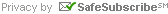For Email Marketing you can trust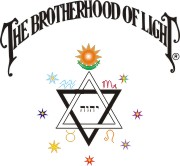## Serial Lesson 111

#### From Course X-2, Progressing the Horoscope, Chapter 2

###### Original Copyright 1934, Elbert Benjamine (a.k.a. C. C. Zain) Copyright 2011, The Church of Light

Calculation Aid:  Correcting Ascendant for Latitude of Birth

Chart Examples:  Majors: Birth Chart 1a    Majors: Birth Chart 316

# Major Progressions of Sun and Angles

IT was Einstein�s Special Theory of Relativity, ollowed to its practical and logical conclusions which led to the discovery of releasing and utilizing atomic energy. And it is this same Special Theory of Relativity followed to its practical and logical conclusions which indicates both how inner-plane energies operate and what can be done to cause them to work more to the individual�s advantage.

As university scientists have conclusively demonstrated that time, distance and gravitation on the inner plane have properties radically different than they have on earth, should we expect inner-plane weather to operate according to the same laws weather operates on earth? Einstein�s Special Theory of Relativity carried to its logical conclusions indicates that inner-plane weather affects the individual not merely according to his inner-plane constitution, but through certain time-space relationships that bring structural changes within his astral body.

Astrological energies constitute the inner-plane weather. How this inner-plane weather affects an individual, however, is not dependent upon any theory; for even as the time, distance and gravitation properties of the inner plane have been determined experimentally by university scientists, so have the properties of inner-plane weather, and how it works to affect individuals, groups, cities, nations and world affairs been determined experimentally, and through statistical studies carried out in the process of astrological research.

One of the outstanding influences of inner-plane weather is that when a person, creature or important event is born, it is born at a time when the inner-plane weather tends to coincide with the inner-plane makeup of that which is then born. Thus does the inner-plane weather at the time of his birth, as mapped by his birth chart, indicate the predisposition of an individual to develop abilities of a certain type. The planetary positions and aspects, whatever they may be, which indicate such a predisposition arc called its Birth-Chart Constants. The statistically ascertained Birth-Chart Constants of 30 different vocations arc set forth in the reference book How to Select a Vocation.

The predispositions indicated by the inner-plane weather conditions at birth never manifest as events or diseases except during those periods when the appropriate thought cells receive commensurate additional energy from inner-plane weather mapped by progressed aspects.

Inner-plane weather consists of astrological vibrations in their infinite variety of combinations. Those mapped by progressed aspects enable planetary vibrations to reach and make active certain groups of thought cells. These thought cells have desires such as were imparted to them when they were formed and as indicated by the aspects of the planets mapping them in the birth chart. Such desires are temporarily altered by the planetary energy reaching them through the inner-plane weather mapped by a progressed aspect. And the additional energy thus reaching the thought cells not only gives them the power to influence the individual�s thoughts and behavior, but it also gives them the psychokinetic activity that enables them to attract events of the kind they desire into his life.

By far the most important inner-plane weather is mapped by major-progressed aspects. Church of Light statistical research covering the lives of many thousands of persons indicates that every important event of life takes place during the period while a major-progressed aspect is present involving planets characteristic of the nature of the event, and which rule the birth-chart house governing the department of life affected. If more than one department of life is pronouncedly affected by the event, at the time it occurs there are always major-progressed aspects involving the ruler of each house governing these various departments of life.

The periods in his life when the individual is likely to experience a specific event, condition or disease toward which he has a predisposition are indicated by certain major-progressed aspects. These progressed aspects mapping inner-plane weather conditions which have been found always to coincide with the given event, condition or disease are called its Progressed Constants. The statistically ascertained Progressed Constants of 20 different events are set forth in the reference book When and What Events Will Happen, and both Birth-Chart Constants and the Progressed Constants of 160 different diseases are set forth in Course XVI, Stellar Healing.

Both the birth-chart position and the progressed position of a planet act as terminals for the reception of planetary energy. Each terminal actually involved in the progressed aspect receives the energy of the progressed aspect in full volume. But unless the progressed aspect is from a major-progressed planet to its birth-chart place—in which case there are only two terminals—each progressed aspect has two other terminals not directly involved in the progressed aspect. Each of these two terminals not directly involved in the progressed aspect receives, through the principle of resonance, one-half as much energy as is received by each terminal directly involved.

It is important to understand that commonly a major-progressed aspect has four terminals because our research has determined that each Major-Progressed Constant of an event or disease is always reinforced by a minor-progressed aspect heavier than from the Moon to one of its four terminals at the time the event occurs or the disease develops; and that each reinforced Major-Progressed Constant of an event or disease is always released by a transit aspect heavier than from the Moon to one of its four terminals at the time the event occurs or the disease develops. And an independent minor-progressed aspect is always released by a transit aspect to one of the birth-chart or major-progressed terminals influenced by the minor-progressed aspect at the time the event takes place.

Before an ephemeris of Pluto was available to permit its aspects to be included, there seemed to be indications that events influenced by progressed aspects of Sun or Mars occasionally took place while the aspect was as much as a degree and half from perfect. But as statistically indicated in C. of L. Astrological Report No. 61, published in the January, 1948, number of “The Rising Star,” in these instances while sometimes the zodiacal aspect was well over the one degree limit, at the same time there was a progressed parallel aspect involving the significant planet which was not over the one degree limit.

The more closely the planets approach the perfect progressed aspect the greater the amount of energy the temporary stellar aerial in the astral body is capable of picking up, radio fashion, and transmitting to the thought cells at its direct and indirect terminals, and the more capable these become of influencing events.

Due to the reinforcement effect of minor-progressed aspects to any of the four terminals of the major-progressed aspect, to the trigger effect of transit aspects to any of the four terminals of the major-progressed aspect, and to the physical environment through which events must come, the important events attracted by major-progressed aspects seldom arrive exactly on the date the progressed aspect is perfect. But other things being equal, they are more apt to arrive close to the date the major-progressed aspect is perfect than while the aspect is farther removed. Therefore, that the time and nature of the important events which will be attracted into the life—unless they are forestalled by precautionary actions—may be estimated in advance, it is essential that the time be known when each major-progressed aspect becomes perfect.

As major progressions arc measured by the ratio of the movements of the planets during one apparent solar day releasing energy which causes the chief structural changes within the astral body that takes place during one astrological year of life, the movements and positions of the planets each four minutes after birth indicate the structural changes that take place within the astral body each corresponding day after birth; the movements and positions of the planets each two hours after birth spread the structural changes so shown over each corresponding month of life after birth; and the movements and the positions of the planets each day after birth indicate the structural changes and events attracted during the corresponding year and time of year of life.

The noon positions (or midnight positions if a midnight ephemeris is used) of the planets as given in the ephemeris must thus represent their major-progressed positions for some year, month and day of calendar time either before or after birth. And as on an average the progressed positions of the planets on the birthday are no closer or farther from making perfect progressed aspects than the progressed positions of the planets for any other day of the year, the calendar date which corresponds to the ephemeris positions of the planets on the day of birth is the most convenient starting point for calculating the calendar date any major-progressed aspect is perfect. Its originator called the calendar date thus found the LIMITING DATE.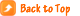### Finding the Limiting Date

The Limiting Date (abbreviated L.D.) is the date in calendar time corresponding to the major-progressed positions of the planets on the day of birth as they are shown in the ephemeris. Convert the EGMT Interval of birth into months and days of calendar time by dividing the hours by 2 and calling the result months, and dividing the minutes by 4 and calling the result days.

If the EGMT Interval of birth is minus, add the calendar interval thus found to the year and month of birth. If the EGMT Interval of birth is plus, subtract the calendar interval thus found from the year, month and day of birth. The L.D. thus found is the calendar starting point from which all major-progressed aspects and positions are calculated. As the birth-chart positions of the planets are calculated for an EGMT Interval, the most convenient time for finding the L.D. is while the chart is being erected. However, should an EGMT Interval on the day preceding or following birth be used in finding the planets, places, this must not be employed in finding the L.D. Instead, the EGMT Interval on the day of birth must be ascertained; for the L.D. must always be calculated from the EGMT Interval on the day of birth. On B. of L. student blanks a space is designated on which to write the L.D. It may be in the year of birth, in the year previous to birth, or in the year following birth. In writing it down be sure to include not only the month and day of month, but also the year in which it falls.

Example 1: Chart la, Jan. 2, 1920, has an EGMT Interval of plus 10h 32m What is the Limiting Date?

10 divided by 2 gives 5 as the month. 32 divided by 4 gives 8 as the days. As the interval is plus, the 5 months, 8 days must be subtracted from Jan. 2, 1920. This gives July 24, 1919, as the L.D. This means that the places of the planets given in the ephemeris for Jan. 3, 1920 (one day after birth) are their major-progressed positions for July 24, 1920. The Map. D. for calendar year 1920 is thus Jan. 3, 1920.

Example 2: Chart 316, Dec. 17, 1920, has an EGMT Interval of minus 3h 30m. What is the Limiting Date?

3 divided by 2 gives 1 month with a remainder of 60 minutes. 60m plus 30m gives 90m. 90 divided by 4 gives 22½ as the days. As the interval is minus, this 1 month, 22½ days must be added to Dec. 17, 1920. This gives Feb. 9, 1921, as the L.D. This means that the positions of the planets on the day of birth, Dec. 17, 1920, are their major-progressed positions for Feb. 9, 1921; and that their positions as shown in the ephemeris for Dec. 18, 1920, are their major-progressed positions for Feb. 9, 1922. Likewise, the positions of the planets in the ephemeris for Dec. 28, 1920, are their major-progressed positions for Feb. 9, 1932. Which means that the Map. D. for 1932 is Dec. 28, 1920.

Example 3: The birth chart of Henry Ford Chapter 5, (Serial Lesson 107), Course X-I, Delineating the Horoscope has an EGMT Interval of plus 7h 56m. What is the Limiting Date?

7 divided by 2 gives 3 as the months, with a remainder of 60m. These added to 56m gives 116m 116 divided by 4 gives 29 as the days. As the Interval is plus, this 3mo 29d must be subtracted from July 30, 1863, which is the date of birth. This gives April 1, 1863, as the L.D. July 31, 1863, represents the major-progressed positions, and is the Map.D. for April 1, 1864; and Aug. 1, 1863, represents the major-progressed positions for April 1, 1865. and is thus the Map.D. for 1865.### Finding the Major Progression Date

Both the L.D. and the Major Progression Date should always be calculated from the date of birth in the ephemeris. Using the day preceding or following birth in the ephemeris is the most common source of error in calculating major progressions. The Major Progression Date (abbreviated Map.D.) is the ephemeris day which shows the major-progressed positions of the planets for the month and day of the Limiting Date, but for some calendar year. To find the Map.D. for any calendar year, count ahead in the ephemeris from the day of birth as many days as complete years have elapsed since the Limiting Date. The ephemeris day so located is the required Map.D. Examples 1, 2 and 3 illustrate the process.### Finding the Midheaven Constant

As explained in Chapter 1 (Serial Lesson 19), the M.C. progresses—by major progression, by minor progression, and by transit progression—exactly the same number of signs, �s and ′s that the Sun progresses through the zodiac during the same time. As the progressed aspects made by the M.C. and Asc. are extremely important—next in importance to those made by the Sun—it is advisable to reduce the work of calculating a series of them, once for all, in each chart by finding the Midheaven Constant.

The Midheaven Constant (abbreviated M.C.C.) is the distance in the chart of birth between the M.C. and the Sun in signs, �s and ′s expressed as a plus or minus, so that when added to the sign �, and ′ occupied by the M.C. the algebraic sum gives the sign, �, and ′ occupied by the Sun. It is found by merely subtracting the smaller zodiacal longitude occupied by birth-chart M.C. or Sun, from the larger zodiacal longitude occupied by birth-chart M.C. or Sun, and placing before the signs, �s, and ′s thus found the proper plus or minus sign.

Then, wherever the M.C. may be by progression—major, minor or transit—algebraically add the sign, �, and ′ it occupies to the M.C.C. and the result is the sign, �, and ′ occupied by the progressed Sun. And wherever the progressed Sun may be, change the sign before the M.C.C. and algebraically add it to the sign, �, and ′ occupied by the progressed Sun and the result is the sign, �, and ′ occupied by the progressed M.C.

Example 4: In chart la, the Sun is 11 Capricorn 16 and the M.C. is 5 Aries 45. From 10S 11� 16′ subtract 1S 5� 45′ and it gives 9S 5� 31′. As to find the position of the Sun the difference so found must be added to the M.C., the M.C.C. is plus 9S 5� 31′.

Example 5: In chart 316, the Sun is 25 Sagittarius 08 and the M.C. is 16 Cancer 45. From 9S 25� 08′ subtract 4S 16� 45′ and it gives 5S 8� 23′. As to find the position of the Sun the difference so found must be added to the M.C., the M.C.C. is plus 5S 8� 23′.

Example 7: In the Henry Ford chart Chapter 5 (Serial Lesson 107), Course X-I, Delineating the Horoscope the Sun is 7 Leo 06 and the M.C. is 12 Virgo 00. From 6S 12� 00′ subtract 5S 7� 06′ and it gives 1S 4� 54′. As to find the position of the Sun the difference so found must be subtracted from the M.C., the M.C.C. is minus 1S 4� 54′.### The Problems of Progressions

The calculations involved in chart erection, major progressions, minor progressions and transit progressions are chiefly the solution of problems in direct proportion such as are taught in grammar school. In each problem (a) : (b) :: (c) : (d).

In thus solving problems in proportion, as the product of the means is equal to the product of the extremes, when the two inner terms are given, multiply one by the other and divide the product by the outer term. When the two outer terms are given, multiply one by the other and divide the product by the inner term. The result is the answer.

Any of these problems in proportion can be solved in four different ways. They can be solved by direct proportion, they can be solved by logarithms, they can be solved with a slide rule, or they can be solved with The Church of Light Chart Calculator.

In mathematically handling proportions involving hours and minutes and �s and ′s, the use of logarithms greatly reduces the labor. Each problem in proportion considered in this book can be worked either by direct proportion, or by logarithms. By either method the letter employed to designate each term of the proportion is as follows:

(a) is the ephemeris daily gain, or gain through some constant interval of time or space.

When only one planet or position is moving, as when a planet is moving to make an aspect with some birth-chart position, the ephemeris daily gain (a) is the daily motion of the planet. When both planets or positions are direct in motion, or when both planets or positions are retrograde in motion, subtract the ephemeris daily motion of the slower moving planet from the ephemeris daily motion of the faster moving planet. The result is (a) the ephemeris daily gain. When one planet or position is direct in motion and the other is retrograde in motion, add the two daily motions. Their sum is (a) the ephemeris daily gain.

(b) is the gain during some selected interval of time or space.

(c) is the constant interval of time or space.

(d) is some selected interval of time or space.

In employing direct proportion to solve problems in progression it is more convenient to work immediately with calendar time. But in employing logarithms it is more convenient to work the problem first in terms of progression time (EGMT Interval), and then convert the result so found into calendar time. Diurnal proportional logarithms such as are to be found in the back of most ephemerides are constructed to solve just such problems in proportion, term (c), which is always 1440 minutes (24 hours), being taken care of by the table.

The advantage of such logarithms, which are almost universally used in erecting birth charts to find how many �s and ′s a planet moves during a given EGMT Interval, is that the logarithm of term (b) can be obtained merely by adding the logarithms of term (a) and (d); and the logarithm of term (d) can be obtained merely by subtracting the logarithm of term (a) from the logarithm of term (b).

To designate the birth-chart position of a planet it has become the custom to use the letter r after the planet, to designate a major-progressed planet to use the letter p after the planet, to designate a minor-progressed planet to use the letter m after the planet, and to designate a transit-progressed planet to use the letter t after the planet.### Finding the Major-Progressed Positions of the Planets on a Given Calendar Date

Find the plus or minus calendar interval in months and days the given date is from the nearest month and day of the L.D. Then find the Map. D. in the ephemeris for the L.D. from which the given calendar date is this number of months and days distant. Convert the calendar interval from the L.D. in that calendar year into EGMT Interval (major progression time) at the rate of each month being equivalent to 2 hours, and each day being equivalent to 4 minutes. If the calendar interval is plus the EGMT Interval thus found is plus; if the calendar interval is minus the EGMT Interval thus found is minus. Use this EGMT Interval on the Map. D. in the ephemeris exactly as if finding the birth-chart positions of the planets for this EGMT Interval on that ephemeris day.

Example 8: Find the major-progressed positions of the planets on Nov. 24, 1949, for chart la. In example I the L.D. is found to be July 24, 1919. Subtracting 7mo 24d from 11mo 24d gives a plus calendar interval of 4mo. Multiplying 4 by 2 gives a plus 8h EGMT Interval. Subtracting 1919 (L.D.) from 1949 gives 30. Adding 30 days to January 2, 1920 (date of birth) gives February 1 as the Map. D. Using the planetary positions on Feb. 1, 1920, and calculating their positions for a plus EGMT Interval of 8 hours, gives their major-progressed positions on November 24, 1949, as shown on below.Correcting Ascendant for Latitude of Birth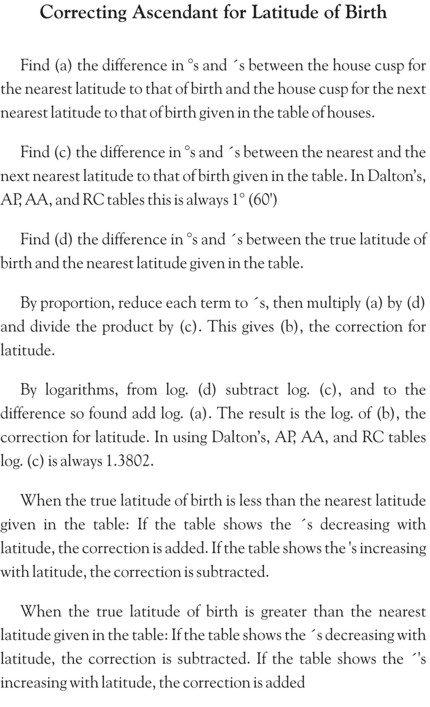Example 9: Find the major-progressed positions of the planets on April 24, 1934, for chart 316. In example 2 the L.D. is found to be Feb. 9, 1921. Subtracting 2mo 9d from 4mo 24d gives a plus 2mo 15d calendar interval. Multiplying 2 by 2 gives 4h. Multiplying 15 by 4 gives 60m, or 1h. There is thus a plus 5h EGMT Interval. Subtracting 1921 (L.D.) from 1934, gives 13. Adding 13 days to Dec. 17, 1920 (date of birth) gives Dec. 30, 1920, as the Map. D. Using the planetary positions on Dec. 30, 1920, and calculating their positions for a plus EGMT Interval of 5h, gives their major-progressed positions on April 24, 1934, as shown on page .### Finding the Major, Minor or Transit-Progressed M.C. on a Given Date

First find the sign, �, and ′ occupied by the progressed Sun on the given calendar date. Change the sign before the M.C.C. and algebraically add the M.C.C. to the sign, �, and ′ occupied by the progressed Sun. The result is the precise progressed M.C.

Example 10: Find major-progressed M.C. for chart la on November 24, 1949. In example 4 the M.C.C. for this chart is shown to be plus 9S 5� 31 ′. Major-progressed Sun on November 24, 1949, is 11 Aquarius 41. Subtracting 9S 5� 31′ from 11S 11� 41′ gives 2S 6� 10′. Thus progressed M.C. is 6 Taurus 10.

Example 11: Find major-progressed M.C. for chart 316 on April 24, 1934. In example 5 the M.C.C. of this chart is shown to be plus 5S 8� 23′. Major-progressed Sun on April 24, 1934, is 8 Capricorn 44. Subtracting 5S 8� 23′ from 10S 8� 44′ gives 5S 0� 21′. Thus progressed M.C. is 0 Leo 21.### Finding the Major, Minor or Transit-Progressed Asc. on a Given Date

In a table of houses look between the two columns within which the progressed M.C. occurs, and find (a) the �s and ′s between the nearest and next nearest Asc. given for the latitude nearest that of birth.

Find (c) the �s and ′s between the nearest and next nearest M.C. given in the table. In Dalton�s, AP, Raphael�s and RC tables this is always 1� (60′).

Find (d) the �s and ′s between the true M.C. and the M.C. given in the table.

By proportion reduce each term to ′s, then multiply ( a ) by ( d ) and divide the product by ( c ). This gives (b), the distance the Asc. is from the nearest Asc. given in the table for the nearest latitude given in the table.

By logarithms, to log. (a) add log. (d), and from the sum so found subtract log. (c). The result is log. (b), the distance the Asc. is from the nearest Asc. given in the table for the nearest latitude given in the table.

If the true M.C. is smaller than the M.C. given in the table, subtract (b) from the nearest Asc. in the table. If the true M.C. is greater than the M.C. given in the table, add (b) to the nearest Asc. in the table.

This gives the Asc. for the latitude given in the table. If the latitude of birth is not precisely that given in the table, use the Correction for Latitude given on page .

Example 12: Find major-progressed Asc. for chart la on November 24, 1949. In example 10 the major-progressed M.C. for this date is shown to be 6 Taurus 10. AP and Raphael�s tables give the Asc. for 6 Taurus as 15 Leo 39 and the Asc. for 7 Taurus as 16 Leo 24. The difference (a) is thus 45′. As 6 Taurus 10 is 10′ more than 6 Taurus, (d) is 10′. (c) is 60′. By proportion, multiplying 45 by 10 gives 450. 450 divided by 60 gives 7½′. By logarithms, the sum of log. (a) 1.5051 and log. (d) 2.1584 is 3.6635. Subtracting log. (c) 1.3802 from 3.6635 gives 2.2833, which is the log. of (b) 7½′. To the Asc. for 6 Taurus, which is 15 Leo 39, we add 8′ (considering the ½ as 1), which, as the table is for the precise latitude of birth, gives the progressed Asc. as 15 Leo 47′.

Example 13: Find major-progressed Asc. for chart 316 on April 24, 1934. In example 11 the major-progressed M.C. for this date is shown to be 0 Leo 21. Dalton�s table gives the Asc. for 0 Leo in latitude 40 as 25 Libra 38, and the Asc. for 1 Leo as 26 Libra 27. The difference (a) is 49′. As 0 Leo 21 is 21′ more than 0 Leo, (d) is 21′. (c) is 60′. By proportion, multiplying 49 by 21 gives 1029. 1029 divided by 60 gives 17. By logarithms, the sum of log. (a) 1.4682 and log. (d) 1.8361 is 3.3043. Subtracting log. (c) 1.3802 from 3.3043 gives log. 1.9241, which is the log. of (b) 17′. To the Asc. for 0 Leo, which is 25 Libra 38, we add 17′, which gives the progressed Asc. for the latitude given in the table as 25 Libra 55.

But as the true latitude of birth is 39� 45′, there is a correction to be made for (d) the 15′ difference in latitude. Under the 0 Leo column the table gives 25 Libra 52 for latitude 39, and 25 Libra 38 for latitude 40, a difference of (a) 14′. (c) is 60′. Following the instructions given on page , by proportion, multiplying(a) 14 by (d) 15 gives 210. Dividing 210 by 60 gives (b) 3½′. By logarithms, subtracting log. (c) 1.3802 from log. (d) 1.9823 gives .6021. Adding log. (a) 2.0122 to .6021 gives 2.6143, which is the log. of (b) 3½′.

As the true latitude is less than the nearest latitude given in the table and the ′s are decreasing with latitude, we add the correction of 3½′ to 25 Libra 55, which (considering the ½ as 1) gives the progressed Asc. as 25 Libra 59.### Finding the Calendar Date on Which a Major-Progressed Aspect Between Planets is Perfect

Find the Map. D. in the ephemeris nearest the ephemeris time the aspect is perfect.

Find (a) the daily gain in �s and ′s of the one planet on the other as indicated on the Map. D. in the ephemeris.

Find (b) the �s and ′s the aspect is from perfect.

In employing proportion (c) is 12 months or 365 days. In employing logarithms (c) is 24h EGMT Interval.

In employing proportion (d) is months and days of calendar time from the L.D. in the calendar year it takes the planets to close the gap (b) and make the perfect aspect.

In employing logarithms (d) is the number of hours and minutes of EGMT Interval on the Map.D. it takes the planets to close the gap (b) and make the perfect aspect. This EGMT Interval must then be converted into its equivalent plus or minus calendar interval at the rate of each 2 hours being equal to 1 month and each 4 minutes equal to 1 day. By either method if the aspect is formed before the positions given in the ephemeris, subtract the calendar interval thus found from the L.D. in the calendar year. If the aspect is formed after the positions given in the ephemeris, add the calendar interval thus found to the L.D. in the calendar year. This gives the calendar date the aspect is perfect.

By proportion, to find (d) multiply (b) by (c) and divide by (a).

By logarithms, to find (d) subtract log. (a) from log.(b).

Example 14: The L.D. for chart la was found to be July 24, 1919. On what date does the Sun make the conjunction with Uranus r by major progression?

Uranus r is 29 Aquarius 03. Turning to the 1920 ephemeris we find the Sun on Feb. 19, 1920, in 29 Aquarius 34, and thus (b) 31 ′ past the perfect aspect. Between Feb. 18 and Feb. 19, 1920, the Sun is moving (a) 61′.

By proportion, multiplying (b) 31 by (c) 12 gives 372. Dividing 372 by (a) 61 gives the calendar interval (d) as 6 6/61 months, or 6mo 3d.

By logarithms, subtract log. (a) 61′, 1.3730 from log. (b) 31′, 1.6670, and it gives log. .2940, which is the log. of (d) 12h 12m. Dividing 12 by 2 gives 6mo. Dividing 12 by 4 gives 3 days.

Counting ahead in the ephemeris from the day of birth, January 2, 1920, we find that Feb. 19 is 48 days later. Adding 48 years to the L.D., July 24, 1919, gives 1967 as the calendar year for Map. D. Feb. 19, 1920. As the aspect was formed before the positions given in the ephemeris on Feb. 19, 1920, we subtract the calendar interval 6mo 3d from July 24, 1967. This gives the date of progressed Sun conjunction Uranus r as January 21, 1967.### Progressing the Sun

Examples of finding the dates of major-progressed aspects of the other planets will be found in Chapters 3 and 4 (Serial Lessons 112 and 113). For precision the calendar date on which each progressed aspect involving the Sun is perfect should be determined in the manner above indicated. But as the daily motion of the Sun varies only from 57′ to 61′, its approximate major progression per month is 1/12 of this, or approximately 5′; and 1′ progression is thus equivalent approximately to 6 days of calendar time. Thus when there is no need for precision it is more convenient to work progressed aspects of the Sun by proportion.

Example 6: Find date on which in chart la major-progressed Sun makes the semisquare with Venus r. Venus is 28 Scorpio 18. The Sun must therefore reach 13 Capricorn 18 to make the semisquare. In example 1, we found the L.D. for this chart to be July 24, 1919. On January 4, 1920, the ephemeris shows the Sun 12 Capricorn 51. It must therefore move 27′ to make the aspect. Dividing 27 by 5 (′s of Sun travel per month) gives 5mo. Multiplying the remaining 2′ by 6 (days the Sun travels in 1′) gives us 12d.

Counting ahead in the ephemeris from the day of birth, January 2, 1920, we find January 4 is 2 days later. Adding 2 years to the L.D. July 24, 1919, gives 1921 as the calendar year for the Map. D. As the aspect was formed after the positions given in the ephemeris, we add the 5mo 12d to July 24, 1921, and it gives the date of Sun semisquare Venus r as January 6, 1922.

When the actual travel of the Sun on the Map. D., 61′, is used, the more precise date obtained by either proportion or logarithms is January 3, 1922. The problem worked out in detail by logarithms is given on page of Chapter 1 (Serial Lesson 19).### Finding the Sign, �, and ′on the M.C. for a Given Asc.

If the table of houses does not give the precise latitude of birth, find the Correction for Latitude as explained on page .

When the true latitude is less than the nearest latitude given in the table: If the table shows the ′s decreasing with latitude, the correction is subtracted. If the table shows the ′s increasing with latitude, the correction is added.

When the true latitude of birth is greater than the nearest latitude given in the table: If the table shows the ′s decreasing with latitude, the correction is added. If the table shows the ′s increasing with latitude, the correction is subtracted.

This gives the Asc. for the nearest latitude given in the table.

Find (a) the �s and ′s between the nearest and the next nearest M.C. in the table. In Dalton�s, AP, Raphael�s and RC tables this is always 1� (60′).

Find (c) the �s and ′s between the nearest and the next nearest Asc. given in the table.

Find (d) the �s and ′s between the true Asc. corrected for the latitude given in the table, and the nearest Asc. for that latitude given in the table.

(b) is the distance the true M.C. is from the nearest M.C. given in the table.

By proportion, to find (b), multiply (a) by (d) and divide the product by (c). By logarithms, add log. (a) to log. (d) and from their sum subtract log. (c).

Example 15: In chart 1a what sign, � and ′ is on the M.C. when progressed Asc. makes the conjunction with Neptune r? To make the conjunction the progressed Asc. must move to 10 Leo 55. Looking in the table of houses for New York we find the nearest Asc. 11 Leo 08, with 30 Aries 00 on the M.C. The next nearest Asc. is 10 Leo 24. The difference (c) is 44′. The difference (d) between 10 Leo 55 and 11 Leo 08 is 13′. By proportion, multiplying (a) 60 by (d) 13 gives 780. Dividing 780 by (c) 44 gives 18′. By logarithms, adding log. (a) 1.3802 to log. (d) 2.0444 gives log. 3.4246. Subtracting log. (c) 1.5149 from 3.4246 gives 1.9097, which is the log. of (b) 18′.

As the Asc. when the aspect is complete is less than the nearest Asc. given in the tables, the 18′ must be subtracted from 30 Aries 00. This gives the progressed M.C. 29 Aries 42.

Example 16: In chart 316 what sign, � and ′ is on the M.C. when progressed Asc. makes the trine with Pluto r? To make the trine with Pluto r the Asc. must move to 8 Scorpio 04. Dalton�s table of houses shows 15 Leo 00 on the M.C. when 7 Scorpio 40 is on the Asc. in lat. 40, and 8 Scorpio 01 on the Asc. in lat. 39. As chart 316 is erected for lat. 39:45, there is a correction to be made for (d) 15′ of latitude. (a) is 21′, the difference between 7 Scorpio 40 and 8 Scorpio 01. Multiplying (a) 21′ by (d) 15′ gives 315. 315 divided by (c) 60′ gives the correction for latitude as 5′. Subtracting this 5′ from 8 Scorpio 04 shows that when 8 Scorpio 04 is on the Asc. in lat. 39 :45, 7 Scorpio 59 is on the Asc. in lat. 40, the lat. given in the table. We need to find, therefore, the sign, �, and ′ on the M.C. when the table shows 7 Scorpio 59 on the Asc. in lat. 40.

In lat. 40 the table shows 7 Scorpio 40 as the nearest Asc. and 8 Scorpio 27 as the next nearest. The difference (c) is 47′. The difference (d) between 7 Scorpio 40 and 7 Scorpio 59 is 19′. By proportion, multiplying (a) 60 by (d) 19 gives 1140. Dividing 1140 by (c) 47 gives 24′. By logarithms, adding log. (a) 1.3802 to log. (d) 1.8796 gives log. 3.2598. Subtracting log. (c) 1.4863 from 3.2598 gives 1.7735, which is the log. of (b) 24′.

As the Asc. when the aspect is complete is more than the nearest Asc. given in the table, the 24′ must be added to 15 Leo 00. This gives the progressed M.C. 15 Leo 24.### Finding the Calendar Date from Major, Minor or Transit-Progressed M.C.

Algebraically add the sign, �, and ′ of the progressed M.C. to the M.C.C. The result is the sign, �, and ′ occupied by the progressed Sun on the sought calendar date. Thus when the sign, �, and ′ occupied by the Asc. when it makes an aspect is determined, the sign, �, and ′ on the M.C. for this Asc. can be ascertained as above explained. And when the sign, �, and ′ occupied by the progressed M.C. when it makes an aspect is determined—as when it makes an aspect to a birth-chart planet—this can be used. In either case, from the M.C. find the sign, �, and ′ occupied by the progressed Sun by algebraically adding to it the M.C.C. Then find the calendar date on which the progressed Sun occupies the sign, �, and ′ so found. This is the precise date on which the progressed Asc. or M.C. makes the aspect, or reaches the given sign, �, and ′ of the zodiac.

Example 17: In chart la, on what date does major-progressed Asc. make the conjunction with Neptune r? In example 15 we found the aspect is perfect when 29 Aries 42 is on the progressed M.C. In example 4 we found the M.C.C. for this chart to be plus 9S 5� 31′. Adding 9S 5� 31′ to 1S 29� 42′ gives the major-progressed position of the Sun 11S 5� 13′. On January 26, 1920, the ephemeris gives the Sun 5 Aquarius 15. This is 2′ past the required position, and as the Sun moves at the rate of 1′ for each 6 days by major progression, this is equivalent to 12 days to be subtracted from the L.D. July 24, in the calendar year, giving July 12. As January 26 is 24 days after birth, we add 24 years to the year of the L.D., 1919. Asc. is conjunction Neptune r July 12, 1943.

Example 18: In chart 316, on what date does major-progressed Asc. make the trine with Pluto r? In example 16 we found the aspect is perfect when 15 Leo 24 is on the M.C. In example 5 we found the M.C.C. for this chart to be 5S 8� 23′. Adding 5S 8� 23′ to 5S 15� 24′ gives the major-progressed position of the Sun as 10S 23� 47′. On January 14, 1921, the ephemeris gives the Sun 23 Capricorn 49. This is 2′ past the required position, and as the Sun moves at the rate of 1′ for each 6 days major progression, this is equivalent to 12 days to be subtracted from the L.D. February 9, in the calendar year, giving (ignoring the 31 days in January) January 27. As January 14 is 28 days after birth, we add the 28 years to the year of the L.D., 1921. Asc. is trine Pluto r January 27, 1949.### Finding the Progressed Zodiacal Motion of Major, Minor or Transit M.C. or Asc.

In a table of houses find the nearest Asc. to that the motion of which is to be ascertained for the latitude nearest that of birth. The difference in Asc. motions between consecutive latitudes is so small that using the motion for the nearest latitude is sufficiently precise.

Find (a) the difference in �s and ′s between the nearest and the next nearest Asc. given in the two columns within which the progressed Asc. is found.

Find (c) the difference in �s and ′s between the nearest and the next nearest M.C. given in the same two columns in the table. In Dalton�s, AP, Raphael�s and RC tables this is always 1� (60′).

Find (d) the daily motion of the Sun in �s and ′s as given in the ephemeris on the Map. D., MED, or Transit Date. This is also the number of �s and ′s traveled by the progressed M.C. during the same progressed interval. It is the daily motion of the M. C. on the Map. D., MED, or Transit Date.

By proportion, multiply (a) by (d) and divide the product by (c). This gives (b). By logarithm, add log. (a) to log. (d), and from the sum subtract log. (c). This gives log. (b). (b) thus found is the �s and ′s the Asc. moves during the same major, minor or transit progression interval moved by the Sun. It is the daily motion of the Asc. on the Map. D. MED, or Transit Date.### Finding the Calendar Date on Which an Aspect Involving Major-Progressed M.C. or Asc. is Perfect

From the daily motion of the M.C. or Asc. on the Map. D., and the daily motion of the planet on the Map. D., find (a) the gain of the one on the other in �s and ′s. If the aspect is from progressed M.C. or Asc. to a birth-chart position, (a) is the daily motion of M.C. or Asc.

Find (b) the �s and ′s the aspect is from perfect. To find (b) first find the sign, �, and ′ occupied by the progressed M.C. or Asc. on the Map. D. Then find the sign, �, and ′ occupied by the progressed planet on the Map. D. The �s and ′s which are less subtracted from the �s and ′s which are greater, gives the �s and ′s the aspect is from perfect.

(c) is 12mo calendar time or 24h EGMT Interval.

With (a), (b) and (c) thus ascertained the date the aspect is perfect is found exactly as in finding the date on which a major-progressed aspect between planets is perfect.

Example 19: In chart la, on what date does major-progressed Sun make the opposition of progressed Asc.? On Feb. 20, 1920, the ephemeris shows Sun 0 Pisces 34. Subtracting the M.C.C. 9S 5� 31′ found in example 4, from 12S 0� 34′ gives the progressed M.C. for this Map. D. 25 Taurus 03. The Asc. when 25 Taurus 03 is on the M.C. as worked from the table of houses for New York is 0 Virgo 31. Progressed Sun, moving faster than progressed Asc. is thus (b) 3′ past the perfect opposition. The Sun on Feb. 20, and therefore the M.C., is moving 60′. The Asc. is moving 48′ while the M.C. moves 60′. The gain (a) of the Sun on the Asc. is the difference between 48′ and 60′ or 12′.

By proportion, multiplying (b) 3 by (c) 12 gives 36. Dividing 36 by (a) 12 gives 3mo. By logarithms, subtracting log. (a) 2.0792 from log. (b) 2.6812 gives log. (d) .6020 which is the log. of 6h. Dividing 6 by 2 gives 3mo. Subtracting the 3mo from the L.D. July 24, in the calendar year, gives April 24. Feb. 20 is 49 days after the day of birth on Jan. 2. To the year of the L.D., 1919, we therefore add 49 years. Progressed Sun is opposition Asc. p April 24, 1968.

Example 20: In chart 316, when does major-progressed Mercury make the sextile with major-progressed Asc.? The Map. D. for 1930 is 9 days after birth, or Dec. 26, 1920. The ephemeris position of Mercury on this date is 22 Sagittarius 23. The position of the Sun on Dec. 26, 1920, is 4 Capricorn 27. In example 5 we found the M.C.C. of this chart to be plus 5S 8� 23′. Subtracting 5S 8� 23′ from 10S 4� 27′ gives the progressed M.C. on the Map. D. 26 Cancer 04. Dalton�s table of houses shows 22 Libra 19 on Asc. in lat. 40 when 26 Cancer 00 is on M.C. Asc. moves 50′ while M.C. moves 60′. Thus when 26 Cancer 04 is on the M.C. 22 Libra 22 is on the Asc. in lat. 40. As explained in example 13 there is a further correction of 3′ to be added which gives the Asc. in lat. 39 :45 as 22 Libra 25.

As the Asc. on Map. D. is 22 Libra 25, and Mercury is 22 Sagittarius 23, the distance aspect is from perfect (b) is 2′.

The Sun on Dec. 26, and therefore the M.C., is moving 61′. To find how far Asc. moves while M.C. moves 61′, as previously explained, multiply (a) 50 by (d) 61. This gives 3050. Then divide by (c) 60, which gives the daily motion of the Asc. (b) as 51′. The daily motion of Mercury on Jan. 26, 1920, is 1� 31′. The daily gain is thus 40′.

By proportion, multiplying (b) 2 by (c) 12 gives 24. Dividing 24 by (a) 40 gives (d) 24/40mo or 18d. By logarithms, subtracting log. (a) 1.5563 from log. (b) 2.8573 gives 1.3010 which is log. (d) 1h 12m. Dividing this 72m by 4 gives 18d. As Mercury is moving faster than the Asc. the aspect is formed after the Map. D. Therefore the 18d must be added to Feb. 9, 1930. Progressed Mercury is sextile Asc. p, Feb. 27, 1930.

### Birth Charts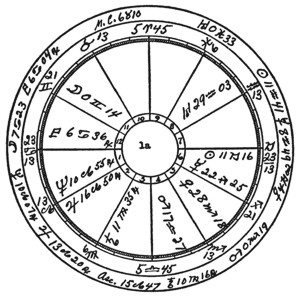Majors: Birth Chart 1a Jan. 2, 1920, 5:32 p.m. EST. 74W. 40:43N. Major progressions in outer circle for Nov. 24, 1949. Asc. semisquare Saturn r, June 25, 1924. Sun sesquisquare Saturn r, January 15, 1935. M.C. opposition Mars p, August 4, 1940. Mercury conjunction Sun p, November 27, 1953. M. C. conjunction Moon r, September 18, 1973. Asc. sextile Mars p, July 7, 1977.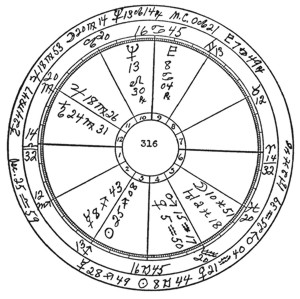Majors: Birth Chart 316 Dec. 17, 1920, 1:30 a.m. MST. 105W. 39 45N Time not recorded: Rectified to 2:10 A.M. Sun sesquisquare Neptune r, April 5, 1924. Asc. semisextile Jupiter r, June 19, 1925. M.C. sextile Saturn r, August 6, 1928. Asc. trine Mars p, August 9, 1929. Mercury inconjunct M.C. p, March 2, 1937. Mercury conjunction Sun p, May 23, 1951.The Sacred TarotArticles and Papers by Elbert BenjamineHistory of the Adepts, Spiritual Ancestors of The Brotherhood of Light LessonsGlobal Astrology Forecasts and ReportsAdditional Articles, Reports, History, DataOrder of the Sphinx Research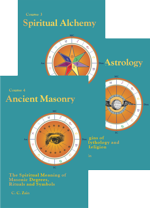Brotherhood of Light 21 Courses eBooks PDF Downloads Kindle iPad, iPhone & Android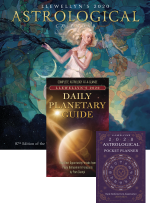2020 Calendar, Daily Planetary Guide, & Pocket Planner